JEE  >  JEE Main Maths Mock Test- 5

# JEE Main Maths Mock Test- 5 - JEE

Test Description

## 25 Questions MCQ Test JEE Main & Advanced Mock Test Series - JEE Main Maths Mock Test- 5

JEE Main Maths Mock Test- 5 for JEE 2023 is part of JEE Main & Advanced Mock Test Series preparation. The JEE Main Maths Mock Test- 5 questions and answers have been prepared according to the JEE exam syllabus.The JEE Main Maths Mock Test- 5 MCQs are made for JEE 2023 Exam. Find important definitions, questions, notes, meanings, examples, exercises, MCQs and online tests for JEE Main Maths Mock Test- 5 below.
Solutions of JEE Main Maths Mock Test- 5 questions in English are available as part of our JEE Main & Advanced Mock Test Series for JEE & JEE Main Maths Mock Test- 5 solutions in Hindi for JEE Main & Advanced Mock Test Series course. Download more important topics, notes, lectures and mock test series for JEE Exam by signing up for free. Attempt JEE Main Maths Mock Test- 5 | 25 questions in 60 minutes | Mock test for JEE preparation | Free important questions MCQ to study JEE Main & Advanced Mock Test Series for JEE Exam | Download free PDF with solutions
 1 Crore+ students have signed up on EduRev. Have you?
JEE Main Maths Mock Test- 5 - Question 1

### The foot of the perpendicular from (- 2, 3) to the line 2x - y - 3 = 0 is

JEE Main Maths Mock Test- 5 - Question 2

### The area of the circle with centre (h,k) and radius a is

JEE Main Maths Mock Test- 5 - Question 3

### [(1+cosθ+i sinθ)/(i+sinθ+i cosθ)]4 = cos nθ + isin nθ, then n=

JEE Main Maths Mock Test- 5 - Question 4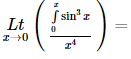JEE Main Maths Mock Test- 5 - Question 5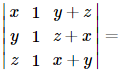JEE Main Maths Mock Test- 5 - Question 6

The differential equation which represents the family of plane curves y=exp. (cx) is

Detailed Solution for JEE Main Maths Mock Test- 5 - Question 6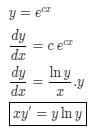JEE Main Maths Mock Test- 5 - Question 7

If the domain of function f(x) = x2 - 6x + 7 is (-∞, ∞), then the range of function is :

JEE Main Maths Mock Test- 5 - Question 8

In the following question, a Statement-1 is given followed by a corresponding Statement-2 just below it. Read the statements carefully and mark the correct answer-
Let a, b, c, p, q be real numbers. Suppose α,β are the roots of the equation x2 + 2px + q = 0 and a, 1/β are the roots of the equation ax2 + 2bx + c = 0, where β2 ∉ {-1, 0, 1}
Statement-1:
(p2-q)(b2-ac)≥0
Statement-2:
b≠pa or c≠qa

Detailed Solution for JEE Main Maths Mock Test- 5 - Question 8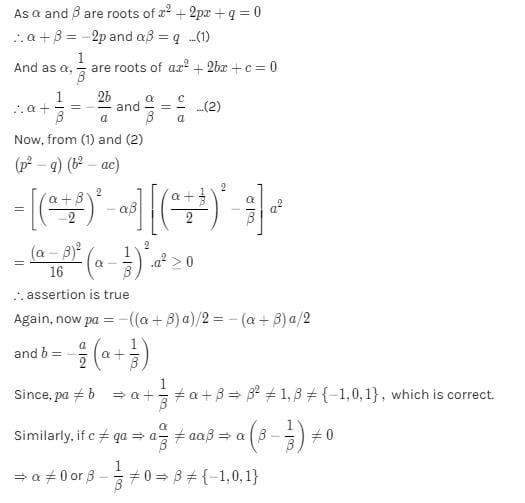JEE Main Maths Mock Test- 5 - Question 9

The angle between the pair of tangents drawn from the point (1,2) to the ellipse 3x2 + 2y2= 5 is

JEE Main Maths Mock Test- 5 - Question 10

(d/dx)[cos(1-x2)2]=

JEE Main Maths Mock Test- 5 - Question 11

In the following question, a Statement of Assertion (A) is given followed by a corresponding Reason (R) just below it. Read the Statements carefully and mark the correct answer-
Assertion(A): ex , loge x are two functions such that each is the image of the other with respect to the line x = y
Reason (R): The inverse of every bijective function is symmetric about the line x = y

JEE Main Maths Mock Test- 5 - Question 12

A square tank of capacity 250 cubic m has to be dug out. The cost of land is Rs 50 per sq.m. The cost of digging increases with the depth and for the whole tank is 400 (depth)2 rupees. The dimensions of the tank for the least total cost are

JEE Main Maths Mock Test- 5 - Question 13

The same roots of 3-4 i are

JEE Main Maths Mock Test- 5 - Question 14

Everybody in a room shakes hands with every body else. The total number of hand shakes is 66. Then the number of persons in the room is

Detailed Solution for JEE Main Maths Mock Test- 5 - Question 14

There are n people in the room
n(n - 1)/2 = 66
n2 - n = 132
n2 - n - 132 = 0
n2 - 12n + 11n - 132 = 0
n(n - 12) + 11(n - 12) = 0
(n + 11) (n - 12) = 0
n = 12, - 11
Hence total no of persons = 12

JEE Main Maths Mock Test- 5 - Question 15

If the sum of the squares of the roots of x2 + px - 3 = 0 is 10, then the values of p =

Detailed Solution for JEE Main Maths Mock Test- 5 - Question 15
Sum of the roots of equation :- α + β = - b/aProducts of the roots of equation :- αβ = c/a squaring on both side sum of roots of equation α^2 +β^2 +2αβ = b^2/a^2 put values in above equation 10 + 2(-3) =b^2 b = +2 or b = -2
JEE Main Maths Mock Test- 5 - Question 16

Three identical dice are rolled. The probability that the same number will appear on each of them, is

JEE Main Maths Mock Test- 5 - Question 17

If A is the single A.M. between two numbers a and b and S is the sum of n A.M.'s between them, then S/A depends upon

JEE Main Maths Mock Test- 5 - Question 18
If the two lines of regression are 5x + 3y = 55 and 7x + y = 45, then the correlation coefficient between x and y is
JEE Main Maths Mock Test- 5 - Question 19

M telegrams are distributed at random over N communication channels (N > M). The probability of the event
A = {not more than one telegram will be sent over any channel} is

Detailed Solution for JEE Main Maths Mock Test- 5 - Question 19

The total no. of ways of distributing M telegrams over N channels = NM
The number of ways of choosing M channels out of N to send one telegram over each channel = NCM
∴ Total no. of ways to send M telegrams over each channel = NCM . M!
∴ Required probubility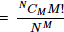JEE Main Maths Mock Test- 5 - Question 20

The equation of a line passing through (-a,0) and form a triangle of area 'T' with coordinates axes, is

*Answer can only contain numeric values
JEE Main Maths Mock Test- 5 - Question 21

If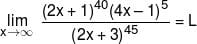then value of L/128 is :-

Detailed Solution for JEE Main Maths Mock Test- 5 - Question 21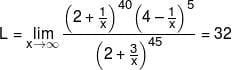*Answer can only contain numeric values
JEE Main Maths Mock Test- 5 - Question 22

If the function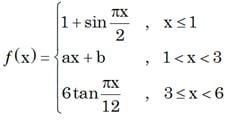is continuous in the interval (–∞, 6) then value of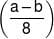is

Detailed Solution for JEE Main Maths Mock Test- 5 - Question 22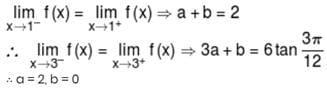*Answer can only contain numeric values
JEE Main Maths Mock Test- 5 - Question 23

Value of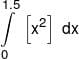is equals to
(where [.] denotes greatest integer function)

Detailed Solution for JEE Main Maths Mock Test- 5 - Question 23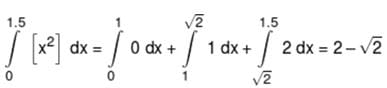*Answer can only contain numeric values
JEE Main Maths Mock Test- 5 - Question 24

If the area bounded by curve x + |y| = 1 and the y-axis is k, then k/4 is equals to

Detailed Solution for JEE Main Maths Mock Test- 5 - Question 24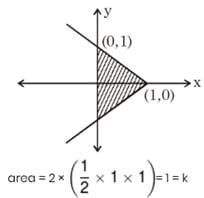*Answer can only contain numeric values
JEE Main Maths Mock Test- 5 - Question 25

If value of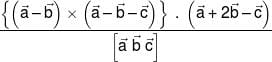is k then k/4 is

Detailed Solution for JEE Main Maths Mock Test- 5 - Question 25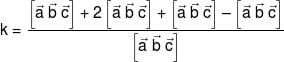k = 3

## JEE Main & Advanced Mock Test Series

1 videos|356 docs|217 tests
Information about JEE Main Maths Mock Test- 5 Page
In this test you can find the Exam questions for JEE Main Maths Mock Test- 5 solved & explained in the simplest way possible. Besides giving Questions and answers for JEE Main Maths Mock Test- 5, EduRev gives you an ample number of Online tests for practice

## JEE Main & Advanced Mock Test Series

1 videos|356 docs|217 tests